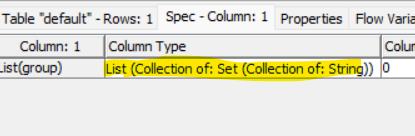# List of sets - Python node

Hello
We have an input table that each row is a list of setsWe want to put run this python code- but how we can read it as a list of sets?
import knime.scripting.io as knio

# This example script simply outputs the node’s input table.

sets = knio.input_tables[‘sets’]

k = len(sets)

distances =
for s1, s2 in combinations(sets, 2):
intersection = s1.intersection(s2)
union = s1.union(s2)
jaccard_distance = 1 - len(intersection) / len(union)
distances.append(jaccard_distance)

diversity = sum(distances) / len(distances)

@malik maybe you can take a look at this example how to transfer Sets between KNIME and Python and also put them to a similar use like you might have tried to do (comparing the ‘distance’ between string item sets):

a

The results would look something like this:

Sets 3 and 5 are deliberately identical2 Likes

My problem, however, is not with inputs but outputs via the knime.scripting.io API.
Here is a snippet of my Python code to output a table list created with the tabulate method in the Tabulate library. Sensitivity and Specificity are scalar values calculated from the confusion matrix cm.

table = [[“Sensitivity val”,sensitivity_cm_val],
[“Specificity val”, specificity_cm_val]]
col_names = [“Metric”, “Value”]
print(accuracy_table)
df_accuracy_table_list = pd.DataFrame(accuracy_table)

knio.output_tables = knio.Table.from_pandas(df_y_val)
knio.output_tables = knio.Table.from_pandas(df_y_pred_val)
knio.output_tables = knio.Table.from_pandas(df_y_pred_prob_val)
knio.output_tables = knio.Table.from_pandas(df_accuracy_table_list)

The first three outputs worked fine; the last one did not. Python complained that I was not calling the DataFrame constructor properly. What did I do wrong?

@Bob_Nisbet can you provide a sample workflow that would reproduce the error without spelling any secrets. I cannot see where and how the “accuracy_table” is being created or what it contains.

The print(accuracy_table) was a typo, it is actually accuracy_table_list.

The problem was with the pandas dataframe constructor. Most languages I know use explicit data typing, while Python data types are implied by the brackets. A scaler is just a list of one member. Therefore, I put square brackets around the scaler values to identify them as lists, and the pandas dataframe constructor worked!
It was a great help to interact with you. It made me think through the problem from another perspective, and that led to the solution. Thank you!

2 Likes

This topic was automatically closed 90 days after the last reply. New replies are no longer allowed.